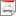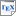[KNP02c] M. Kwiatkowska, G. Norman and A. Pacheco. Model Checking CSL Until Formulae with Random Time Bounds. In H. Hermanns and R. Segala (editors), Proc. PAPM/PROBMIV'02, volume 2399 of Lecture Notes in Computer Science, pages 152-168, Springer. July 2002. [ps.gz] [pdf] [bib] Downloads:ps.gz (115 KB)pdf (408 KB)bib Notes: The original publication is available at link.springer.com. Links: [Google] [Google Scholar] [CiteSeer] Abstract. Continuous Time Markov Chains (CTMCs) are widely used as the underlying stochastic process in performance and dependability analysis. Model checking of CTMCs against Continuous Stochastic Logic (CSL) has been investigated previously by a number of authors [2, 4, 13]. CSL contains a time-bounded until operator that allows one to express properties such as "the probability of 3 servers becoming faulty within 7.01 seconds is at most 0.1". In this paper we extend CSL with a random time-bounded until operator, where the time bound is given by a random variable instead of a fixed real-valued time (or interval). With the help of such an operator we can state that the probability of reaching a set of goal states within some generally distributed delay while passing only through states that satisfy a certain property is at most (at least) some probability threshold. In addition, certain transient properties of systems which contain general distributions can be expressed with the extended logic. We extend the efficient model checking of CTMCs against the logic CSL developed in  to cater for the new operator. Our method involves precomputing a family of coefficients for a range of random variables which includes Pareto, uniform and gamma distributions, but otherwise carries the same computational cost as that for ordinary time-bounded until in . We implement the algorithms in Matlab and evaluate them by means of a queueing system example.